### Home > CALC > Chapter 3 > Lesson 3.2.2 > Problem3-62

3-62.
1. Hanah wrote this derivative function: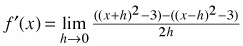Homework Help ✎

1. What is f(x) ?

2. What is f ′(x) ? (Note--Avoid the algebra by using the Power Rule.)

3. Use your slope function to find f ′(0) and f ′( l ).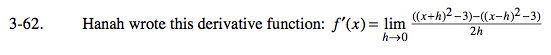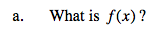Hanah's (symmetrical difference) definition of the derivative;

$f'(x)=\lim_{h\rightarrow 0}\frac{f(x+h)-f(x-h)}{2h}$

f(x) = x2 − 3

Find f '(x), which is also the value of the limit.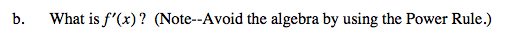Power Rule
if f(x) = xn
then f '(x) = nxn − 1

f(x) = x2 − 3
f '(x) = __________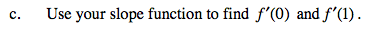Evaluate.